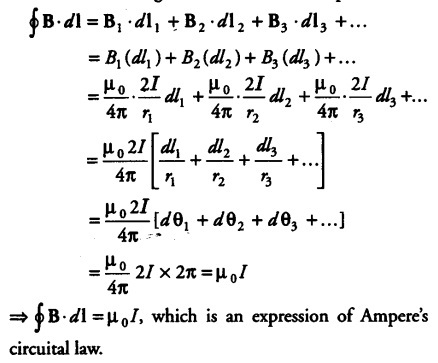# Explain how Biot-Savart’s law

Explain how Biot-Savart’s law enables one to express the Ampere’s circuital law in the integral form, viz.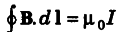where, I is the total current passing through the surface.Consider any arbitrary closed path perpendicular to the plane of paper around a long straight conductor XY carrying current from X to Y, lying in the plane of paper. Let the closed path be made of large number of small elements, where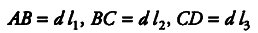be the angles subtended by the various elements at point O through which conductor is passing. Then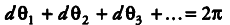Suppose these small elements AB, BC, CD are
small circular arcs of radii r1, r2, r3,… respectively.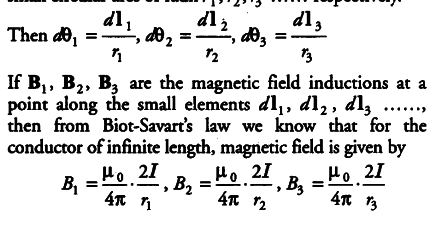In case of each element, the magnetic field induction B and current element vector d\ are in the same direction. Line integral of B around closed path is